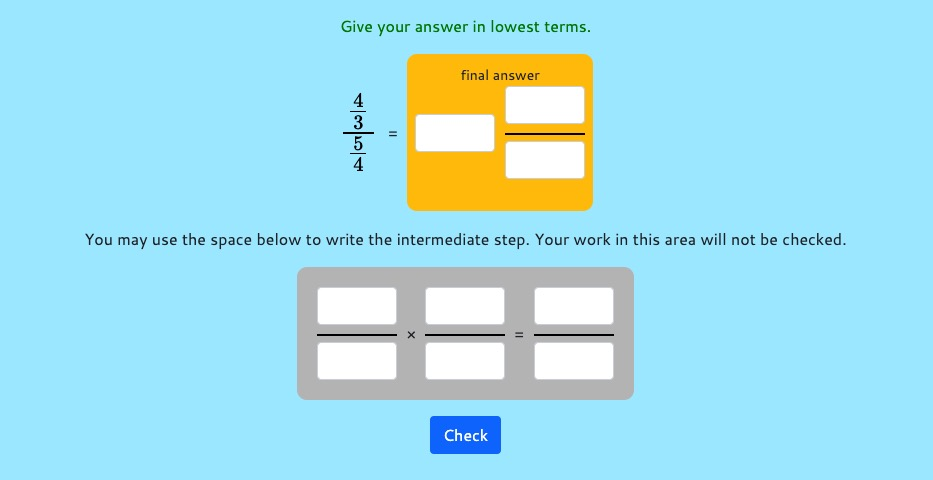MM Practice
×
Multiplication
Division
Place Value
Fractions & Decimals
Measurement
Statistics & Probability
Pre-Algebra
Money
Kindergarten
Geometry

# Simplify Complex FractionsOnline practice for grades 7-9

Practice simplifying complex fractions in this online activity.

Complex fractions are essentially fraction divisions, so all you need to do is divide the two numbers, using the regular rule for fraction division (multiply by the reciprocal of the divisor).

These online exercises give you a special working space for writing out the step where you use fraction multiplication. Your work in this work area will not be checked.

You can choose to include mixed numbers or not. The script will automatically include fractions and whole numbers in the problems.

The options include one divided by unit fraction, unit fraction divided by a whole number, easy divisions done with mental math, fraction divided by a fraction, and mixed number divisions.

Note: ALL answers have to be given as mixed numbers, when possible. In other words, your answer cannot be left as an improper fraction.

Screenshot:OR
Practice for a set time: min

Allow my comment to be posted on this site